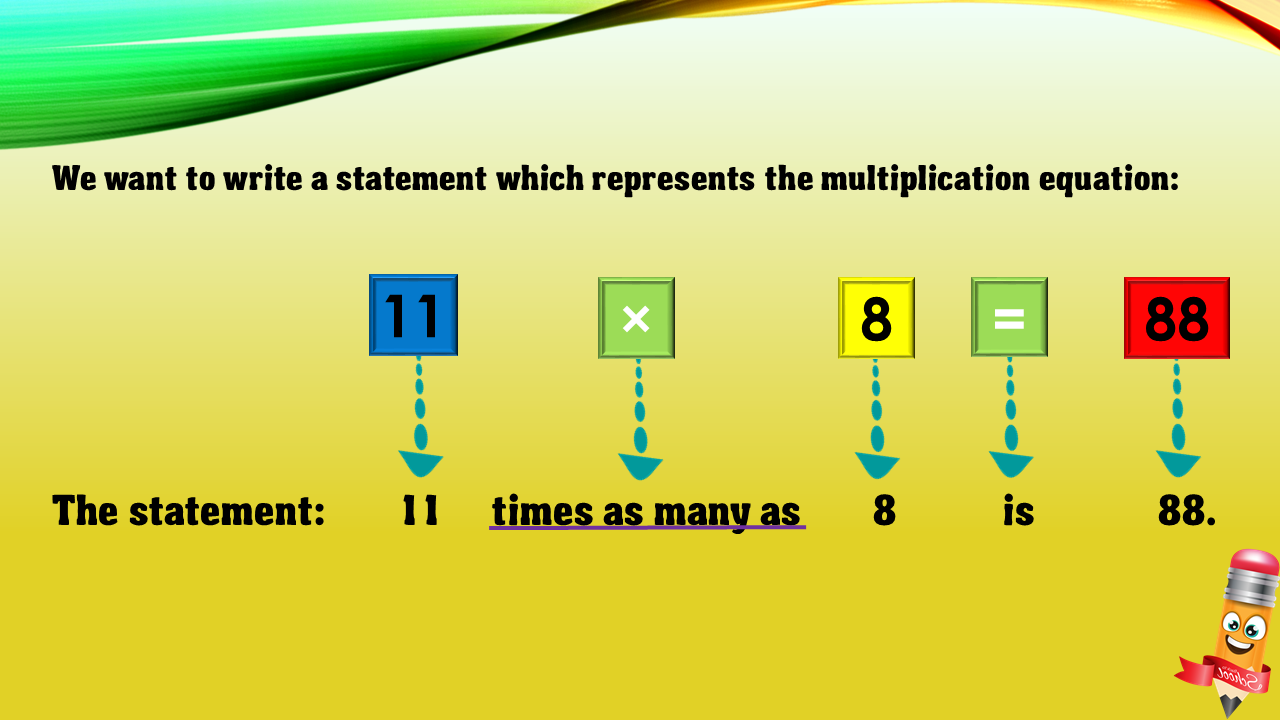1
visibility

Choose one statement which represents the equation:
11 × 8 = 88 ?

• A

11 more than 8 is 88.

• B

11 times as many as 8 is 88.

• C

8 less than 88 is 11.

• D

8 times as many as 88 is 11.